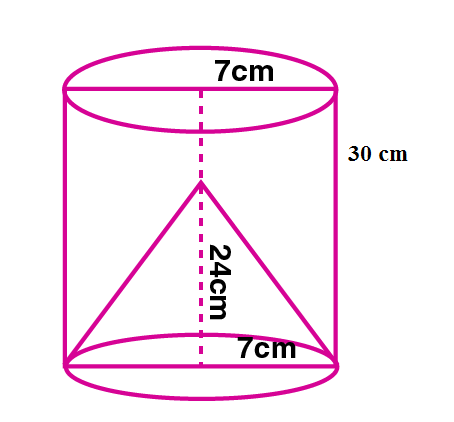Newbie

# From a solid cylinder of height 30 cm and radius 7 cm, a conical cavity of height 24 cm and of base radius 7 cm is drilled out. Find the volume and the total surface of the remaining solid.

• 2

An Important Question of M.L Aggarwal book of class 10 Based on Mensuration Chapter for ICSE BOARD.
Here height and radius of a solid cylinder is given, if a conical cavity of height and base radius given is drilled out. Find the volume and the total surface of the remaining solid.
This is the Question Number 12, Exercise 17.4 of M.L Aggarwal.

Share

1.This answer was edited.Given height of the cylinder, H = 30 cm

Radius of the cylinder, r = 7 cm

Height of cone, h = 24 cm

Radius of cone, r = 7 cm

Slant height of the cone, l = √(h2+r2)

= √(242+72)

= √(576+49)

= √(625)

= 25 cm

Volume of the remaining solid = Volume of the cylinder – Volume of the cone

= r2H – (1/3)r2h

= r2(H- h/3)

= (22/7)×72×(30-24/3)

= (22×7)×(30-8)

= (154)×(22)

= 3388 cm3

Volume of the remaining solid is 3388 cm3.

Total surface area of the remaining solid = Curved surface area of cylinder + surface area of top of the cylinder+ curved surface area of the cone

Total surface area of the remaining solid = 2rH + r2 + rl

= r(2H + r + l)

=(22/7)×7(2×30 + 7+ 25)

= 22×(60+32)

= 22×92

= 2024 cm2

Hence the total surface area of the remaining solid is 2024 cm2.

• 4